# Exam Mission

Exam Information| Govt Jobs.| Exam Preparation | Exam Study Material |Exam Date|Material PDF

1. Experiment:
An operation which can produce some well-defined outcomes is called an experiment.
2. Random Experiment:
An experiment in which all possible outcomes are know and the exact output cannot be predicted in advance, is called a random experiment.
Examples:
1. Rolling an unbiased dice.
2. Tossing a fair coin.
3. Drawing a card from a pack of well-shuffled cards.
4. Picking up a ball of certain colour from a bag containing balls of different colours.
Details:
1. When we throw a coin, then either a Head (H) or a Tail (T) appears.
2. A dice is a solid cube, having 6 faces, marked 1, 2, 3, 4, 5, 6 respectively. When we throw a die, the outcome is the number that appears on its upper face.
3. A pack of cards has 52 cards.
It has 13 cards of each suit, name Spades, Clubs, Hearts and Diamonds.
Cards of spades and clubs are black cards.
Cards of hearts and diamonds are red cards.
There are 4 honours of each unit.
There are Kings, Queens and Jacks. These are all called face cards.
3. Sample Space:
When we perform an experiment, then the set S of all possible outcomes is called the sample space.
Examples:
1. In tossing a coin, S = {H, T}
2. If two coins are tossed, the S = {HH, HT, TH, TT}.
3. In rolling a dice, we have, S = {1, 2, 3, 4, 5, 6}.
4. Event:
Any subset of a sample space is called an event.
5. Probability of Occurrence of an Event:
Let S be the sample and let E be an event.
Then, E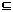S.P(E) = n(E) . n(S)
6. Results on Probability:
1. P(S) = 1
2.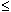P (E)1
3. P(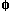) = 0
4. For any events A and B we have : P(A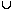B) = P(A) + P(B) - P(A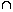B)
5. If A denotes (not-A), then P(A) = 1 - P(A).